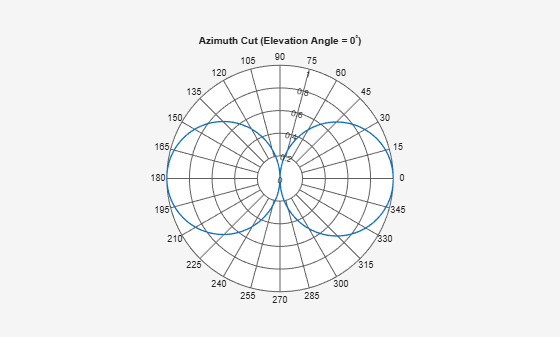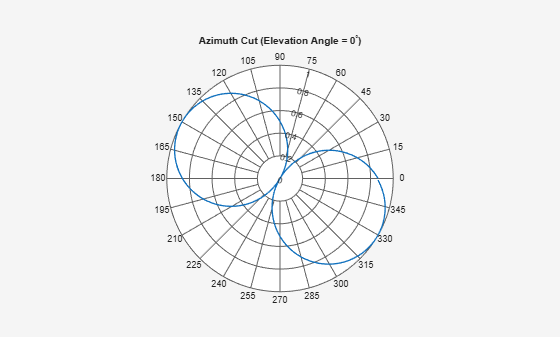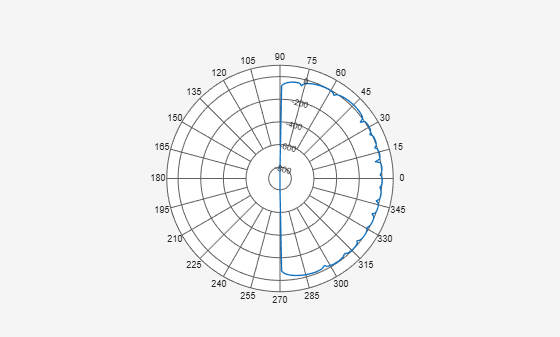# replace

Class: polarpattern

Replace polar plot data with new data

## Syntax

``replace(p,data)``
``replace(p,angle,magnitude)``

## Description

example

````replace(p,data)` removes all data from polar plot, `p` and adds new data based on real amplitude values, `data`. ```

example

````replace(p,angle,magnitude)` removes all the current data and adds new data sets of angle vectors and corresponding magnitude matrices to the polar plot, `p`.```

## Input Arguments

expand all

Polar plot, specified as a scalar handle.

Antenna or array data, specified as one of the following:

• A real length-M vector, where M contains the magnitude values with angles assumed to be $\frac{\left(0:M-1\right)}{M}×{360}^{\circ }$ degrees.

• A real M-by-N matrix, where M contains the magnitude values and N contains the independent data sets. Each column in the matrix has angles taken from the vector $\frac{\left(0:M-1\right)}{M}×{360}^{\circ }$ degrees. The set of each angle can vary for each column.

• A real N-D array, where N is the number of dimensions. Arrays with dimensions `2` and greater are independent data sets.

• A complex vector or matrix, where `data` contains Cartesian coordinates ((x,y) of each point. x contains the real part of `data` and y contains the imaginary part of `data`.

When data is in a logarithmic form such as dB, magnitude values can be negative. In this case,`polarpattern` plots the lowest magnitude values at the origin of the polar plot and highest magnitude values at the maximum radius.

Set of angles, specified as a vector in degrees.

Set of magnitude values, specified as a vector or a matrix. For a matrix of magnitude values, each column is an independent set of magnitude values and corresponds to the same set of angles.

## Examples

expand all

Plot cosine pattern in polar coordinates. Specify a cosine antenna pattern from 0° to 360° in azimuth at 0° elevation. Then, plot the antenna pattern using `polarpattern`.

Create the pattern.

```az = [0:360]; a = abs(cosd(az));```

Plot the polar pattern of the antenna for an azimuth cut at 0° elevation.

`P = polarpattern(a,'TitleTopTextInterpreter','tex','TitleTop','Azimuth Cut (Elevation Angle = 0^{\circ})');`Replace this plot with a rotated cosine pattern.

```a = abs(cosd(az + 30.0)); replace(P,a);```Create a 15-element ULA of cosine antennas with elements spaced one-half wavelength apart. Plot the directivity of the array at 20 GHz.

Note: This example runs only in R2016b or later. If you are using an earlier release, replace each call to the function with the equivalent `step` syntax. For example, replace `myObject(x)` with `step(myObject,x)`.

```fc = 20.0e9; c = physconst('Lightspeed'); lam = c/fc; angs = [-180:1:180]; antenna = phased.CosineAntennaElement('FrequencyRange',[1.0e9,100.0e9],... 'CosinePower',[2.5 2.5]); array = phased.ULA('Element',antenna,'NumElements',15,'ElementSpacing',lam/2); a = pattern(array,fc,angs,0); P = polarpattern(angs,a);```Then, steer the array to 45° and, using the `replace` method, replace the existing polar plot with the steered array directivity.

```steervec = phased.SteeringVector('SensorArray',array,'PropagationSpeed',c,... 'IncludeElementResponse',true); sv = steervec(fc,[45;0]); a1 = pattern(array,fc,angs,0,'Weights',sv); replace(P,angs,a1);```## Version History

Introduced in R2016a